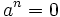# Nilpotent element

## Definition

### Symbol-free definition

An element in a commutative unital ring is termed nilpotent if some positive power of it is$0$.

### Definition with symbols

An element$a$ in a commutative unital ring$R$ is termed nilpotent if there exists an integer$n$ such that$a^n = 0$.